# Math Geometry VocabularyPage 2

#### WATCH ALL SLIDES

A PLANE (no, not the one that flies!) is a flat surface that goes on forever in all directions.

Imagine sitting on a row boat in the middle of the ocean. No matter which way you look…all you see is water…forever.

Slide 13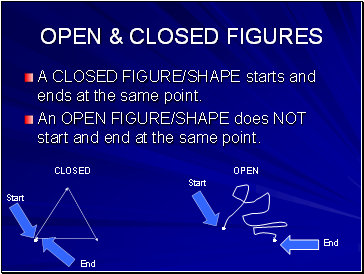## Open & closed figures

A CLOSED FIGURE/SHAPE starts and ends at the same point.

An OPEN FIGURE/SHAPE does NOT start and end at the same point.

CLOSED

OPEN

Start

End

Start

End

Slide 14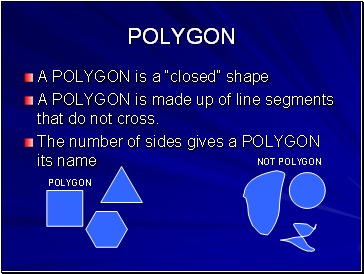## Polygon

A POLYGON is a “closed” shape

A POLYGON is made up of line segments that do not cross.

The number of sides gives a POLYGON its name

POLYGON

NOT POLYGON

Slide 15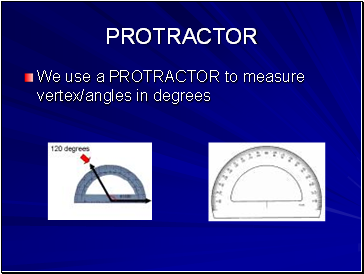## Protractor

We use a PROTRACTOR to measure vertex/angles in degrees

Slide 16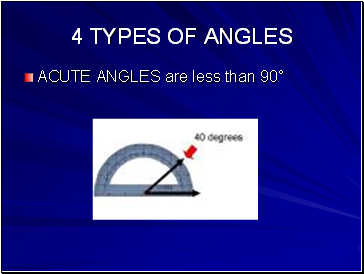## 4 types of angles

ACUTE ANGLES are less than 90°

Slide 17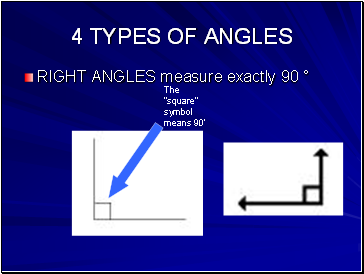4 TYPES OF ANGLES

RIGHT ANGLES measure exactly 90 °

The “square” symbol means 90’

Slide 184 TYPES OF ANGLES

OBTUES ANGLES are greater than 90 ° but less than 180 °

Slide 19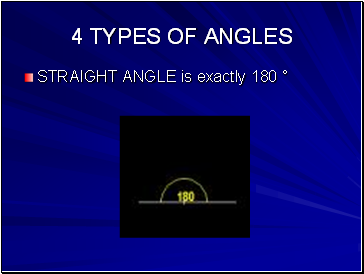4 TYPES OF ANGLES

STRAIGHT ANGLE is exactly 180 °

Slide 20## Angles

When you “name” an angle. The vertex/angle “letter/number” goes in the center of the label

A

B

C

If I wanted to know the measurement of Angle A…I would ask:” What is the measurement for BAC?” (Notice A is in the center)

Slide 21The End

Once you study all the “fancy words”, Geometry is very easy to understand…so STUDY!

Go to page:
1  2